# Grids#

Datashader renders data into regularly sampled arrays, a process known as rasterization, and then optionally converts that array into a viewable image (with one pixel per element in that array).

In some cases, your data is already rasterized, such as data from imaging experiments, simulations on a regular grid, or other regularly sampled processes. Even so, the rasters you have already are not always the ones you need for a given purpose, having the wrong shape, range, or size to be suitable for overlaying with or comparing against other data, maps, and so on. Datashader provides fast methods for “regridding”/”re-sampling”/”re-rasterizing” your regularly gridded datasets, generating new rasters on demand that can be used together with those it generates for any other data types. Rasterizing into a common grid can help you implement complex cross-datatype analyses or visualizations.

In other cases, your data is stored in a 2D array similar to a raster, but represents values that are not regularly sampled in the underlying coordinate space. Datashader also provides fast methods for rasterizing these more general rectilinear or curvilinear grids, known as quadmeshes as described later below. Fully arbitrary unstructured grids (Trimeshes) are discussed separately.

## Re-rasterization#

First, let’s explore the regularly gridded case, declaring a small raster using Numpy and wrapping it up as an xarray DataArray for us to re-rasterize:

```import numpy as np, datashader as ds, xarray as xr
from datashader import transfer_functions as tf, reductions as rd

def f(x,y):
return np.cos((x**2+y**2)**2)

def sample(fn, n=50, range_=(0.0,2.4)):
xs = ys = np.linspace(range_, range_, n)
x,y = np.meshgrid(xs, ys)
z   = fn(x,y)
return xr.DataArray(z, coords=[('y',ys), ('x',xs)])

da = sample(f)
```

Here we are declaring that the first dimension of the `DataArray` (the rows) is called `y` and corresponds to the indicated continuous coordinate values in the list `ys`, and similarly for the second dimension (the columns) called `x`. The coords argument is optional, but without it the default integer indexing from Numpy would be used, which would not match how this data was generated (sampling over each of the `ys`).

DataArrays like `da` happen to be the format used for datashader’s own rasterized output, so you can now immediately turn this hand-constructed array into an image using `tf.shade()` just as you would for points or lines rasterized by datashader:

```tf.shade(da)
```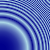## Interpolation (upsampling)#

So, what if we want a larger version? We can do that with the Datashader `Canvas.raster` method. Note that `Canvas.raster` only supports regularly spaced rectilinear, 2D or 3D `DataArray`s, and so will not accept the additional dimensions or non-separable coordinate arrays that xarray allows (for which see Quadmesh, below). Also see the Quadmesh docs below if you want to use a GPU for processing your raster data, because the Datashader `Canvas.raster` implementation does not yet support GPU arrays.

Assuming you are ready to use `Canvas.raster`, let’s try upsampling with either nearest-neighbor or bilinear interpolation (the default):

```tf.Images(tf.shade(ds.Canvas().raster(da, interpolate='linear'),  name="linear interpolation (default)"),
```
 linear interpolation (default)nearest-neighbor interpolation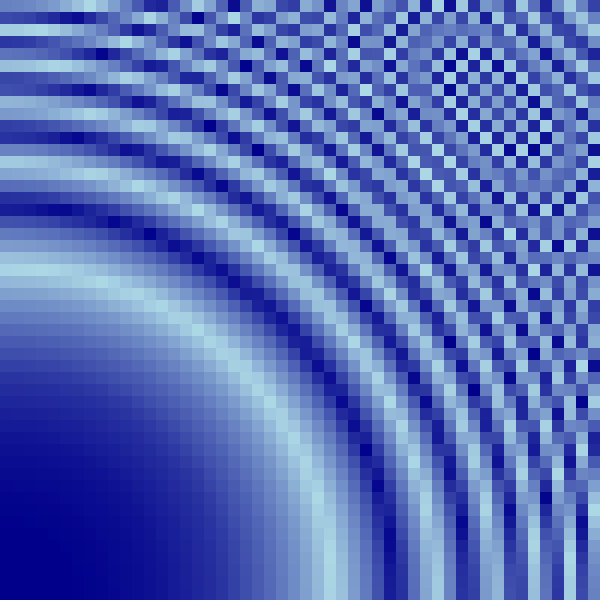As you can see, linear interpolation works well for smoothly varying values, like those in the lower left of this function, but doesn’t help much for regions close to or beyond the sampling limits of the original raster, like those in the upper right.

We can choose whatever output size we like and sample the grid over any range we like, though of course there won’t be any data in regions outside of the original raster grid:

```tf.shade(ds.Canvas(plot_height=200, plot_width=600, x_range=(-2,5), y_range=(-0.1,0.4)).raster(da))
```## Aggregation (downsampling)#

The previous examples all use upsampling, from a smaller to a larger number of cells per unit distance in X or Y. Downsampling works just as for points and lines in Datashader, supporting various aggregation functions. These aggregation functions determine the result when more than one raster grid cell falls into a given pixel’s region of the plane. To illustrate downsampling, let’s first render a 500x500 version of the above 50x50 array:

```da2 = sample(f, n=500)
```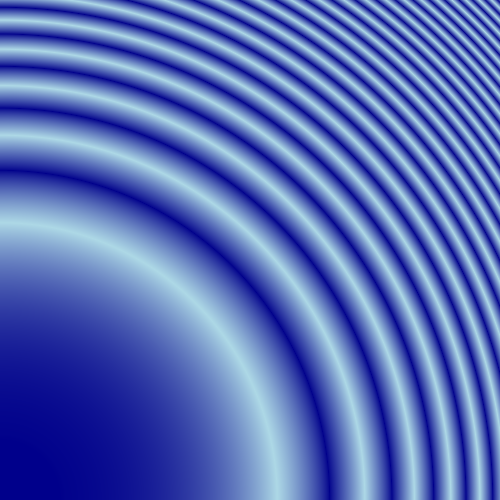You can see that the version we upsampled to this size from the 50x50 samples is similar to this, but this one is much smoother and more accurately represents the underlying mathematical function, because it has sufficient resolution to avoid aliasing in the high-frequency portions of this function (towards the upper right).

Now that we have this larger array, we can downsample it using a variety of aggregation functions:

```cvs = ds.Canvas(plot_width=150, plot_height=150)
 mean downsampling (default)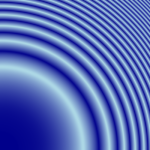min downsampling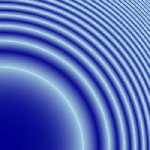max downsampling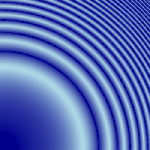mode downsampling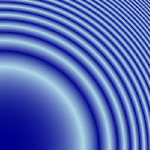std downsampling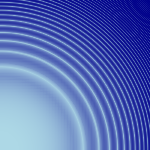Here the default downsampling function `mean` renders a faithful size-reduced version of the original, with all the raster grid points that overlap a given pixel being averaged to create the final pixel value. The `min` and `max` aggregation functions take the minimum or maxiumum, respectively, of the values overlapping each pixel, and you can see here that the `min` version has larger light-blue regions towards the upper right (with each pixel reflecting the minimum of all grid cells it overlaps), while the `max` version has larger dark-blue regions towards the upper right. The `mode` version computes the most common value that overlaps this pixel (not very useful for floating-point data as here, but important for categorical data where `mean` would not be valid; in that case you can also use `first` or `last` to take the first or last value found for a given pixel). The `std` version reports the standard deviation of the grid cells in each pixel, which is low towards the lower left where the function is smooth, and increases towards the upper right, where the function value varies significantly per pixel (i.e., has many samples in the original grid with different values).
```da3 = sample(f, n=500, range_=(0,3))